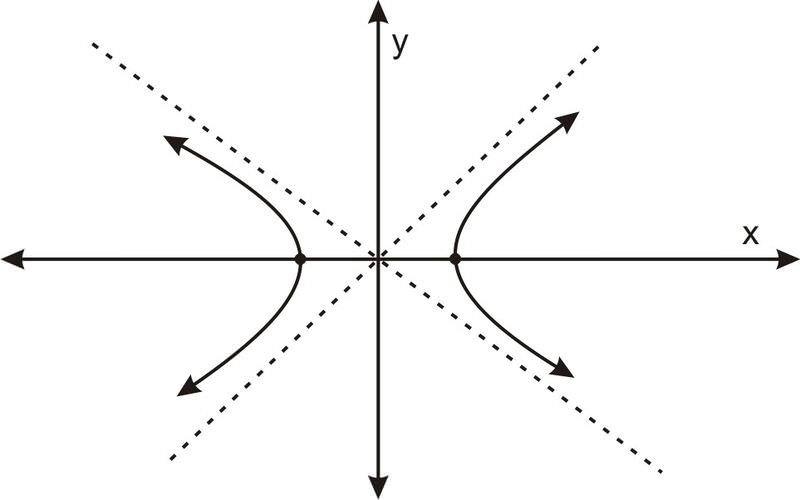Wednesday, November 29

# Hyperbola

0
1072Geometry is a branch of mathematics that deals with sizes, shapes, positions angles, and dimensions. Apollonius introduced the conic section with many important discoveries that have remained unsurpassed for eighteen centuries. Hyperbola is an important topic in the conic section. It is also known as a mirror image of the parabola. We will discuss the main properties and previous year questions related to it in this article.

As far as the JEE exam is concerned, the conic section is an important topic. The locus of points in such a way that the distance to each focus is a constant greater than one is called a hyperbola. It is the set of all points in a plane, the difference of whose distances from two fixed points in the plane is constant. It consists of two curves, each with a vertex and a focus. The transverse axis is the axis that crosses through both vertices and foci. The conjugate axis is the axis perpendicular to it. The plane cuts the two nappes of the cone, which leads to the formation of two disjoint open curves.## Standard equation

The standard equation is given by (x2/a2) – (y2/b2) = 1. Here a denotes the distance from the centre and the vertex. Also b = a2(e2-1).

### Important terms

• Foci: The two fixed points are called foci.
• Centre: The intersection point of the transverse and conjugate axis. It is the midpoint of the line which joins the two foci.
• Transverse axis: A line passing through the foci is the transverse axis. It is also called the major axis.
• Conjugate axis: A line perpendicular to the transverse axis passing through the centre of is the conjugate axis.
• Vertex: The intersection with the transverse axis at two points can be termed as the vertices.
• Focal chord: A chord passing through a focus is called a focal chord.
• Double ordinate: Chord perpendicular to the transverse axis is called a double ordinate.
• Latus Rectum: Focal chord which is perpendicular to the transverse axis is called latus rectum.

### Important formulas

1. Eccentricity (e): e2 = 1 + (b2/a2). e is always greater than or equal to 1.
2. Length of latus rectum = 2b2/a
3. Focii: S = (ae, 0) & S′ = (−ae, 0)
4. Directrix: x = (a/e), x = (−a / e)
5. Vertices: A = (a, 0) & A’ = (-a, 0)

Students are recommended to practise previous year questions so that they can improve their knowledge in the topic. Practicing Hyperbola JEE Previous Year Solved Questions will help students to learn the topic clearly. Following is an example of the JEE question.

Example

If the latus rectum of a hyperbola is 8 and eccentricity is 3 /√5, then find its equation.

Solution:

Given latus rectum = 8

eccentricity , e = 3 /√5

2b2/a = 8

b2 = 4a …(i)

3/√5 = √(1 + b2/a2)

3/√5 = √(a2+b2) / a

Squaring both sides, we get

9/5 = (a2+b2) / a2 .. (ii)

Substitute (i) in (ii)

9/5 = (a2+4a) / a2

9a2 = 5a2+20a

4a2 – 20a = 0

4a(a-5) = 0

a = 5 . (since a cannot be zero)

b2 = 4*5 = 20

Substitute a and b in ( x2/a2) – (y2/b2) = 1

We get

( x2/25) – (y2/20) = 1